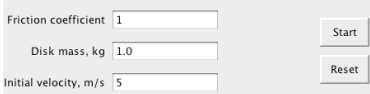# how to get in game numerical input

Hello i would like to know how to be able to enter data into a text box in unity. the following image shows what im talking aboutIn the image there are 3 input boxes for numbers, i would like to know how to create this in unity and take the numerical data from it .

Any help appreciated

Thanking You

I assume you mean at runtime… For this you can use a GUI.TextField:

``````var stringToEdit : String = "3456";

function OnGUI () {
// Make a text field that modifies stringToEdit.
stringToEdit = GUI.TextField (Rect (10, 10, 200, 20), stringToEdit, 25);
}
``````

you would then need to parse the string to an int or float, if you are after numerical data…

something like

`````` var theInt : int = parseInt (stringToEdit);
``````